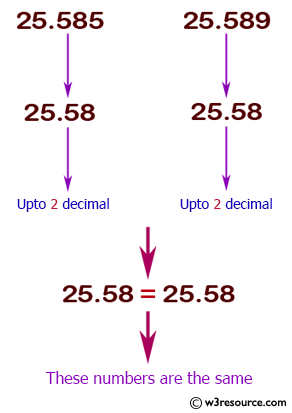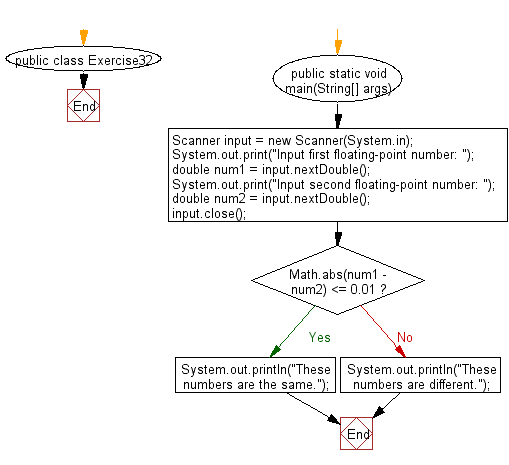﻿ Java exercises: Accepts two floating­point numbers and checks whether they are the same up to two decimal places - w3resource# Java Conditional Statement Exercises: Accepts two floating­point numbers and checks whether they are the same up to two decimal places

## Java Conditional Statement: Exercise-32 with Solution

Write a Java program that accepts two floating­point numbers and checks whether they are the same up to two decimal places.

Test Data
Input first floating­point number: 1235
Input second floating­point number: 2534

Pictorial Presentation:Sample Solution:

Java Code:

``````import java.util.Scanner;
public class Exercise32 {

public static void main(String[] args) {
Scanner input = new Scanner(System.in);
System.out.print("Input first floating­point number: ");
double num1 = input.nextDouble();
System.out.print("Input second floating­point number: ");
double num2 = input.nextDouble();
input.close();

if (Math.abs(num1 - num2) <= 0.01) {
System.out.println("These numbers are the same.");
}
else {
System.out.println("These numbers are different.");
}
}
}
```
```

Sample Output:

```Input first floating­point number: 1235
Input second floating­point number: 2534
These numbers are different.
```

Flowchart:Java Code Editor:

Previous: Accepts three numbers and prints
Next: Java Array exercises

What is the difficulty level of this exercise?

﻿

## Java: Tips of the Day

How to split a String by space?

You can use the whitespace regex:

```str = "Hello I'm your String";
String[] splited = str.split("\\s+");
```

This will cause any number of consecutive spaces to split your string into tokens.

Ref: https://bit.ly/3xk42If